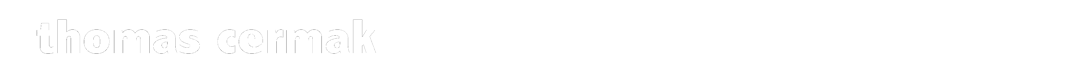2010

V O L P O N E

STEPHAN ZWEIG

NACH BEN JONSON

C O R V I N O

REGIE MICHAEL HELLE

STAATSTHEATER DARMSTADT

T I M O N   V O N   A T H E N

WILLIAM SHAKESPEARE

L U C I U S ,   C A P H I S ,

2 .   S E N A T O R   &   C H O R

REGIE MICHAEL HELLE

STAATSTHEATER DARMSTADT

D I E   H E I L I G E   J O H A N N A

V O N

D E N   S C H L A C H T H Ö F E N

BERTOLT BRECHT

P I E R P O N T   M A U L E R

REGIE KATHARINA KREUZHAGE

THEATER DER SATDT AALEN

M I N N AV O N   B A R N H E L M

G. E. LESSING

W I R T

REGIE INGMAR OTTO

THEATER DER STADT AALEN

D E R   H A U P T M A N N

V O N   K Ö P E N I C K

CARL ZUCKMAYER

D R .   O B E R M Ü L L E R

& VON SCHLETTOW

REGIE WOLF D. SPRENGER

ERNST-DEUTSCH-THEATER HAMBURG

2011

D I E   K U N S T

D E S   F A L L E N

CHRISTIAN NUSSBAUMEDER

J O S E P H

REGIE JENS POTH

STAATSTHEATER DARMSTADT

J U D   S Ü S S

PAUL KORNFELD

U L R I C H

REGIE HERRMANN SCHEIN

STAATSTHEATER DARMSTADT

T U R A N D O T

FRIEDRICH SCHILLER

A L T O U M

REGIE INGMAR OTTO

THEATER DER STADT AALEN

D E R   Z A U B E R E R   V O N   O Z

L. FRANK BAUM

Z A U B E R E R   V O N   O Z

REGIE INA A. KEPPEL

STAATSTHEATER DARMSTADT

f o t o :   b a r b a r a   a u m ü l l e rj o s e p h   -   k u n s t   d e s   f a l l e n s